``````
``````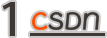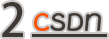• 单击：提供命令行界面（CLI）。

• tqdm：在终端上显示一个优雅的进度栏。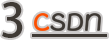``````pip install pipenv # if not installed yet
pipenv install --dev
pipenv shell
``````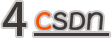• ‍video_path：本地计算机中视频的路径。

• image_path：输出图像的路径和文件名。

• step / -s（可选）：用于获取帧的步骤。这是一个可选参数，默认值为1。

``````@cli.command()@click.argument("video_path", nargs=1, type=str)
@click.argument("image_path", nargs=1, type=str)
@click.option(
"--step",
"-s",
default=1,
type=int,
show_default=True,
help="Step used to get the frames.",
)
def local_video(video_path, image_path, step):
"""Apply the long exposure algorithm to a local video."""
long_exposure = LongExposure(video_path, image_path, step)
long_exposure()
``````

``````python src/long_exposure.py local-video video.mp4 image.png -s 5
``````

``````python src/long_exposure.py --help
``````

• video：本地计算机中的视频路径。

• output_image_path：必须在其中保存输出图像的路径/文件名。

• step：用于忽略某些帧的步骤（默认为1）。这对于长视频非常有用。‍

``````class LongExposure:
def __init__(self, video, output_image_path, step=1):
self.video = video
self.output_image_path = output_image_path
self.step = step
``````

`````` @staticmethod
def averager():
"""Calculate the average using a clojure."""
count = 0
total = 0.0

def average(value):
nonlocal count, total
count += 1
total += value

return average
``````

• 加载视频；

• 通过视频帧进行迭代以计算平均图像（针对每个颜色通道）；

• 合并颜色通道；以及

• 将映像保存在磁盘上。

``````def __call__(self):
logging.info("Processing video %r with step %r", self.video, self.step)

# Open a pointer to the video file
stream = cv2.VideoCapture(self.video)

# Get the total frames to be used by the progress bar
total_frames = int(stream.get(cv2.CAP_PROP_FRAME_COUNT))

r, g, b = None, None, None
r_avg, g_avg, b_avg = self.averager(), self.averager(), self.averager()

for count in tqdm(range(total_frames)):
# Split the frame into its respective channels

if count % self.step == 0:
# Get the current RGB
b_curr, g_curr, r_curr = cv2.split(frame.astype("float"))
r, g, b = r_avg(r_curr), g_avg(g_curr), b_avg(b_curr)

# Merge the RGB averages together and write the output image to disk
avg = cv2.merge([b, g, r]).astype("uint8")
logging.info("Saving image as %r", self.output_image_path)
cv2.imwrite(self.output_image_path, avg)

# Release the stream pointer
stream.release()
``````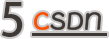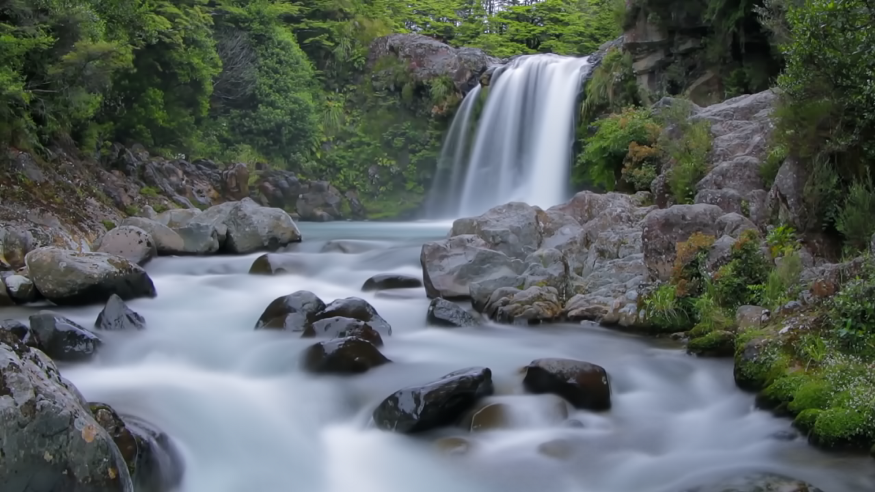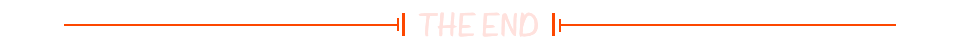``````

☞爷青结？诺基亚贝尔实验室官宣转让Plan 9版权！☞底层I/O性能大PK：Python/Java被碾压，Rust有望取代C++
☞大咖面对面｜人工智能时代，我们应该思考的3个问题
``````20年前，《新程序员》创刊时，我们的心愿是全面关注程序员成长，中国将拥有新一代世界级的程序员。20年后的今天，我们有了新的使命：助力中国IT技术人成长，成就一亿技术人！